Examples using MPI_GATHER, MPI_GATHERV

## Examples using MPI_GATHER, MPI_GATHERV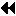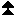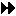Up: Gather Next: Scatter Previous: Gather

```

Gather 100 ints from every process in group to root. See figure
3
.

MPI_Comm comm;
int gsize,sendarray;
int root, *rbuf;
...
MPI_Comm_size( comm, &gsize);
rbuf = (int *)malloc(gsize*100*sizeof(int));
MPI_Gather( sendarray, 100, MPI_INT, rbuf, 100, MPI_INT, root, comm);

```

```

Previous example modified -- only the root allocates memory for the

MPI_Comm comm;
int gsize,sendarray;
int root, myrank, *rbuf;
...
MPI_Comm_rank( comm, myrank);
if ( myrank == root) {
MPI_Comm_size( comm, &gsize);
rbuf = (int *)malloc(gsize*100*sizeof(int));
}
MPI_Gather( sendarray, 100, MPI_INT, rbuf, 100, MPI_INT, root, comm);

```
figure=mycoll-fig2.ps,width=3.50in
Figure 3: The root process gathers 100  ints from each process in the group.

```

Do the same as the previous example, but use a derived datatype.  Note that
the type cannot be the entire set of  gsize*100 ints since type matching
is defined pairwise between the root and each process in the gather.

MPI_Comm comm;
int gsize,sendarray;
int root, *rbuf;
MPI_Datatype rtype;
...
MPI_Comm_size( comm, &gsize);
MPI_Type_contiguous( 100, MPI_INT, &rtype );
MPI_Type_commit( &rtype );
rbuf = (int *)malloc(gsize*100*sizeof(int));
MPI_Gather( sendarray, 100, MPI_INT, rbuf, 1, rtype, root, comm);

```

```

Now have each process send 100 ints to root, but place each set (of 100)
stride ints apart at receiving end. Use  MPI_GATHERV
and the  displs
argument to achieve this effect. Assume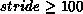.
See figure 4
.

MPI_Comm comm;
int gsize,sendarray;
int root, *rbuf, stride;
int *displs,i,*rcounts;

...

MPI_Comm_size( comm, &gsize);
rbuf = (int *)malloc(gsize*stride*sizeof(int));
displs = (int *)malloc(gsize*sizeof(int));
rcounts = (int *)malloc(gsize*sizeof(int));
for (i=0; i<gsize; ++i) {
displs[i] = i*stride;
rcounts[i] = 100;
}
MPI_Gatherv( sendarray, 100, MPI_INT, rbuf, rcounts, displs, MPI_INT,
root, comm);

Note that the program is erroneous if stride < 100.
```
figure=mycoll-fig3.ps,width=3.50in
Figure 4: The root process gathers 100  ints from each process in the group, each set is placed  stride ints apart.

```

Same as Example Examples using  MPI_GATHER,  MPI_GATHERV
on the receiving side, but send the
100 ints from the 0th column of a
100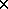150 int array, in C.  See figure 5
.

MPI_Comm comm;
int gsize,sendarray;
int root, *rbuf, stride;
MPI_Datatype stype;
int *displs,i,*rcounts;

...

MPI_Comm_size( comm, &gsize);
rbuf = (int *)malloc(gsize*stride*sizeof(int));
displs = (int *)malloc(gsize*sizeof(int));
rcounts = (int *)malloc(gsize*sizeof(int));
for (i=0; i<gsize; ++i) {
displs[i] = i*stride;
rcounts[i] = 100;
}
/* Create datatype for 1 column of array
*/
MPI_Type_vector( 100, 1, 150, MPI_INT, &stype);
MPI_Type_commit( &stype );
MPI_Gatherv( sendarray, 1, stype, rbuf, rcounts, displs, MPI_INT,
root, comm);

```
figure=mycoll-fig4.ps,width=4.00in
Figure 5: The root process gathers column  0 of a 100\$ x \$150 C array, and each set is placed  stride ints apart.

```

Process i sends (100-i) ints from the ith column of a
100150 int array, in C.  It is received into a buffer with stride,
as in the previous two examples. See figure 6
.

MPI_Comm comm;
int gsize,sendarray,*sptr;
int root, *rbuf, stride, myrank;
MPI_Datatype stype;
int *displs,i,*rcounts;

...

MPI_Comm_size( comm, &gsize);
MPI_Comm_rank( comm, &myrank );
rbuf = (int *)malloc(gsize*stride*sizeof(int));
displs = (int *)malloc(gsize*sizeof(int));
rcounts = (int *)malloc(gsize*sizeof(int));
for (i=0; i<gsize; ++i) {
displs[i] = i*stride;
rcounts[i] = 100-i;     /* note change from previous example */
}
/* Create datatype for the column we are sending
*/
MPI_Type_vector( 100-myrank, 1, 150, MPI_INT, &stype);
MPI_Type_commit( &stype );
/* sptr is the address of start of "myrank" column
*/
sptr = &sendarray[myrank];
MPI_Gatherv( sptr, 1, stype, rbuf, rcounts, displs, MPI_INT,
root, comm);

Note that a different amount of data is received from each process.
```
figure=mycoll-fig5.ps,width=4.00in
Figure 6: The root process gathers 100-i ints from column  i of a 100\$ x \$150 C array, and each set is placed  stride ints apart.

```

Same as Example Examples using  MPI_GATHER,  MPI_GATHERV
, but done in a different way at the sending end.
We create a datatype that causes the correct striding at the
sending end so that that we read a column of a C array.
A similar thing was done in Example Examples
,
Section Examples
.

MPI_Comm comm;
int gsize,sendarray,*sptr;
int root, *rbuf, stride, myrank, disp, blocklen;
MPI_Datatype stype,type;
int *displs,i,*rcounts;

...

MPI_Comm_size( comm, &gsize);
MPI_Comm_rank( comm, &myrank );
rbuf = (int *)malloc(gsize*stride*sizeof(int));
displs = (int *)malloc(gsize*sizeof(int));
rcounts = (int *)malloc(gsize*sizeof(int));
for (i=0; i<gsize; ++i) {
displs[i] = i*stride;
rcounts[i] = 100-i;
}
/* Create datatype for one int, with extent of entire row
*/
disp = 0;       disp = 150*sizeof(int);
type = MPI_INT; type = MPI_UB;
blocklen = 1;   blocklen = 1;
MPI_Type_struct( 2, blocklen, disp, type, &stype );
MPI_Type_commit( &stype );
sptr = &sendarray[myrank];
MPI_Gatherv( sptr, 100-myrank, stype, rbuf, rcounts, displs, MPI_INT,
root, comm);

```

```

Same as Example Examples using  MPI_GATHER,  MPI_GATHERV
at sending side, but
at receiving side we make the
stride between received blocks vary from block to block.
See figure 7
.

MPI_Comm comm;
int gsize,sendarray,*sptr;
int root, *rbuf, *stride, myrank, bufsize;
MPI_Datatype stype;
int *displs,i,*rcounts,offset;

...

MPI_Comm_size( comm, &gsize);
MPI_Comm_rank( comm, &myrank );

stride = (int *)malloc(gsize*sizeof(int));
...
/* stride[i] for i = 0 to gsize-1 is set somehow
*/

/* set up displs and rcounts vectors first
*/
displs = (int *)malloc(gsize*sizeof(int));
rcounts = (int *)malloc(gsize*sizeof(int));
offset = 0;
for (i=0; i<gsize; ++i) {
displs[i] = offset;
offset += stride[i];
rcounts[i] = 100-i;
}
/* the required buffer size for rbuf is now easily obtained
*/
bufsize = displs[gsize-1]+rcounts[gsize-1];
rbuf = (int *)malloc(bufsize*sizeof(int));
/* Create datatype for the column we are sending
*/
MPI_Type_vector( 100-myrank, 1, 150, MPI_INT, &stype);
MPI_Type_commit( &stype );
sptr = &sendarray[myrank];
MPI_Gatherv( sptr, 1, stype, rbuf, rcounts, displs, MPI_INT,
root, comm);

```
figure=mycoll-fig6.ps,width=4.00in
Figure 7: The root process gathers 100-i ints from column  i of a 100\$ x \$150 C array, and each set is placed  stride[i] ints apart (a varying stride).

```

Process i sends  num ints from the ith column of a
100150 int array, in C.  The complicating factor is that
the various values of  num are not known to  root, so a
separate gather must first be run to find these out.  The data is
placed contiguously at the receiving end.

MPI_Comm comm;
int gsize,sendarray,*sptr;
int root, *rbuf, stride, myrank, disp, blocklen;
MPI_Datatype stype,types;
int *displs,i,*rcounts,num;

...

MPI_Comm_size( comm, &gsize);
MPI_Comm_rank( comm, &myrank );

/* First, gather nums to root
*/
rcounts = (int *)malloc(gsize*sizeof(int));
MPI_Gather( &num, 1, MPI_INT, rcounts, 1, MPI_INT, root, comm);
/* root now has correct rcounts, using these we set displs[] so
* that data is placed contiguously (or concatenated) at receive end
*/
displs = (int *)malloc(gsize*sizeof(int));
displs = 0;
for (i=1; i<gsize; ++i) {
displs[i] = displs[i-1]+rcounts[i-1];
}
*/
rbuf = (int *)malloc(gsize*(displs[gsize-1]+rcounts[gsize-1])
*sizeof(int));
/* Create datatype for one int, with extent of entire row
*/
disp = 0;       disp = 150*sizeof(int);
type = MPI_INT; type = MPI_UB;
blocklen = 1;   blocklen = 1;
MPI_Type_struct( 2, blocklen, disp, type, &stype );
MPI_Type_commit( &stype );
sptr = &sendarray[myrank];
MPI_Gatherv( sptr, num, stype, rbuf, rcounts, displs, MPI_INT,
root, comm);

```Up: Gather Next: Scatter Previous: Gather